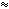From: Headmaster Hello, How many digits has the number 10100 if we write it in binary form? We have three responses for you Use the fact that 210 is approximately 103. (In fact, 210 = 1024.) That makes 2330 in the neighborhood of 1099. Thus, the answer to your question is that 10100 requires about 333 binary digits. If you want to be more accurate you will have to work harder. Chris Using a calculator: 23.321= 9.993569... 23.322 = 10.0005... So, 10100> (23.321)100 = 2332.1 And, 10100< (23.322)100 = 2332.2 So, in the binary expansion of of 10100, there will be a digit (0 or 1) for each of the following places: 20, 21, 22, ..., 2332 Hence, there are 333 digits Paul The number of digits of a number is revealed when you look at the logarithm of the number using a base equal to the number of possible digits. For example, 243 (in normal decimal notation - base 10) has three digits. When we take the logarithm in base 10, we see log10 2432.39. We ignore the decimal portion and add one to the whole number and get 3 digits. Let's try this using binary. 243 in base ten is 11110011 in base two (binary). This is frequently written 24310 = 111100112. Now take the logarithm in base two: log2 2437.92. Again, we drop the .92 and then add one to the seven and our method gives the correct 8 digits. So your question can be answered if you add one to the whole number portion of log2 10100 . Remember to use the shortcuts that logarithms permit with exponents. Hope this helps, Stephen La Rocque.